Blog Post

# Using the Time In & Time Out Calculations On Your Forms

Posted by Brett LongCalculating billable hours is a truth in any business. You want to know how many hours were worked, how much money was made and how much of that money will end up in your pocket – those are questions to which everyone wants the answers!

Now, whether the calculating is done using a pen and paper, a calculator, or your brain (if you are math-inclined), it goes without saying that the repetitive calculations are tedious and time consuming.

In order to go about making the calculating of this information quick and effortless, all you need is to know the correct formulas to put into the “Expression” field in the calculation question.

Here’s a scenario:

Richard works as a supervisor in a large plumbing company that dispatches plumbers to people’s homes at all hours of the day and night.

While calculating billable hours for all the plumbers can be a lengthy process, Richard uses Device Magic’s Mobile Forms – which means the hard work is done for him!

When he first began using it, all he had to do was create a form that contained the “Hours Worked” calculation question to send out to the plumbers’ mobile devices.

Below you can see the Expression Editor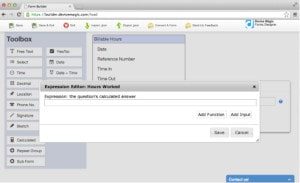Here is the Function Wizard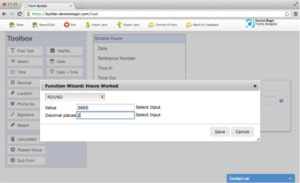And here is the Select Input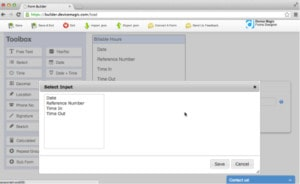Lastly, below is the completed formula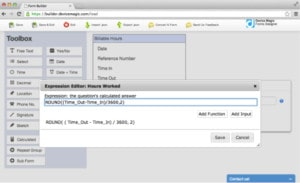Let’s now turn our attention to Simon.

Simon is a plumber who works under Richard. When he arrives at his destination (as assigned to him by Richard), he takes out his mobile device, opens the form, and inserts the current time into the “Time In” question.

He begins his work.

And then, when he’s done, he takes out his mobile device, opens the form, and inserts the time into the “Time Out” question.

Automatically the “Hours Worked” are calculated (as seen below).Simon lastly submits the form, and Richard receives the submission, which looks like this: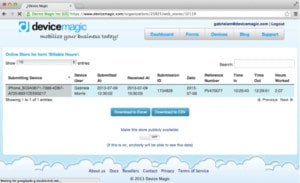Formulas:

Time_Out – Time_In = the answer in seconds (when a time answer is subtracted from another, the result is the difference in seconds)

(Time_Out – Time_In) / 60 = the answer in minutes

ROUND((Time_Out – Time_In) / 60, 0) = the answer in minutes, rounded to the nearest whole number (the “0” indicates it will round to the nearest whole number)

(Time_Out – Time_In) / 3600 = the answer in hours

ROUND((Time_Out – Time_In) / 3600, 2) = the answer in hours, rounded to 2 decimal places

***

Bear in mind that you can either fill in the formulas by using the “Function Wizard” and the “Select Input” in the Expression Editor, or you can copy and paste the formulas listed above.

Alternatively, to bypass any effort at all, here is the JSON file for you to download.

← Back to Blog